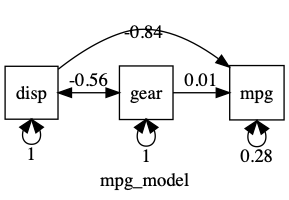# umx!

## umxModify & umxCompare

### Why make a new model when you can update one you already have?

This tutorial covers using `umxModify`.

In `umx`, models can be modified. Want to free a path? Go ahead - no need to start from scratch. You can add, or remove most things, and re-run, and compare in one call.

`umxModify` is a convenience function to add, set, or drop parameters, re-name, and re-run a model.

For what follows, we’ll need a model, so:

``````manifests = c("mpg", "disp", "gear")

m1 = umxRAM("mpg_model",data = mtcars, type = "cov",
umxPath(c("disp", "gear"), to = "mpg"),
umxPath("disp", with = "gear"),
umxPath(var = manifests)
)
``````

Now show a summary.

``````umxSummary(m1, std = TRUE)
plot(m1, std= TRUE)
``````## Modify a model

Fundamentally, modeling is in the service of understanding causality and we do that primarily via model comparison: Better theories fit the data better than do worse theories.

So, “do more gears give better miles per gallon”? In graph terms, this is asking, “is the path from to gear to mpg significant?” There are two ways to test this with `umx`.

### 1. Overwriting a path with OpenMx’s mxModel function

Using OpenMx’s mxModel function, new paths over-write existing paths. So adding a new path, with value fixed to zero will accomplish our goal:

``````m2 = mxModel(m1, umxPath("gear", to = "mpg", fixedAt = 0))
m2 = mxRun(m2)
``````

You can then compare the two models

``````umxCompare(m1, m2)
``````

### 2. Use umxModify() and labels

The easiest and most powerful way to modify models is with umx’s `umxModify` function.

For example, this one-liner drops the path “gear_to_mpg”, tests the effect, and returns the updated model:

``````m2 = umxModify(m1, update = "gear_to_mpg", name = "drop effect of gear", comparison = TRUE)
``````

And viola:

name Estimate SE
disp_to_mpg -0.041 0.005
mpg_with_mpg 9.911 2.478
disp_with_disp 14880.775 3720.133
disp_with_gear -49.215 17.914
gear_with_gear 0.527 0.132

χ²(1) = -0.03, p = 1.000; CFI = 1.021; TLI = 1.063; RMSEA = 0

Model EP Δ -2LL Δ df p AIC Δ AIC Compare with Model
mpg_model 6       -0.047875 0
mpg_model 5 0.0145592 1 0.904 -2.033316 -1.9854408 mpg_model

### More advanced options in umxModify

1. Pattern matching with regex So far we’ve seen update by matching a label:
``````fit2 = umxModify(fit1, update = "Cs", name = "newModelName")
``````

A powerful additional feature of `umxModify` is regular expressions. These let you drop collections of paths by matching patterns.

So, to drop all paths with labels matching “gear_to_” followed by any characters, you could say:

``````m2 = umxModify(m1, regex = "gear_to_.*", name = "drop all paths from gear", comparison = TRUE)
``````

nb: This is particularly powerful updating twin models with many paths to drop.

Regular expressions are very powerful.

``````fit2 = umxModify(fit1, update = "C[sr]", regex = TRUE, name = "drop_Cs_andCr")
``````

You can also update a model by passing in new objects to be added to the model, like a CI request:

``````fit2 = umxModify(fit1, update = mxCI("Cs"), name = "withCI")
``````

Also, we seen that you can request the model comparison summary at the same time:

To run `umxCompare`() and report on the old and new models, just set “comparison = TRUE”

### free and value

By default, matched labels will be dropped, i.e., “free = FALSE value = 0”

To free (instead of fix) a variable, set `free = TRUE`.

To set the value of the matched paths to value X (instead of the default 0), set `value = X`.

To update only parameters that are already free, set `freeToStart = TRUE`. This defaults to NA - i.e, state is ignored.

### more than one thing

Most parameters can take more than one input. To drop two paths, just offer up two labels in `update`.

``````m2 = umxModify(m1, update = c("path1", "path2"), name = "drop 2 paths")
``````

### tryHard

If you have a hard model to fit, you might want the optimizer to try extra hard. Just set tryHard = “yes”:

``````m2 = umxModify(m1, regex = "gear_to_.*", name = "drop paths from gear", tryHard = "yes")
``````

### update a label

If you want to update a label, you can do so as follows: In this case, setting to labels to a single newlabel

``````m2 = umxModify(m1, update = c("gear_to_mpg", "disp_to_mpg"), newlabels = "inputs_to_mpg", name = "equated_paths", tryHard = "yes")
``````

note: this updates the model in raw units: standardized effects will likely differ. Best to work in standardized data for this sort of change.

### Confidence Intervals

As in `mxRun`, you can set `intervals` = TRUE to run confidence intervals (see mxRun)

``````m2 = umxModify(m1, update = "gear_to_mpg", name = "no gear", intervals = TRUE)
``````

note 1: For this to work, there have to be mxCIs in your model. See the post on mxConfint to learn more about confidence intervals.

note 2: SEs are often quicker and easier and are shown in the summary.